### Practice Set 2.1 Pythagoras Theorem Class 10th Mathematics Part 2 MHB Solution

Practice Set 2.1
1. Identify, with reason, which of the following are Pythagorean triplets. (i) (3, 5, 4)…
2. In figure 2.17, ∠MNP = 90°, seg NQ ⊥ seg MP, MQ = 9,QP = 4, find NQ.…
3. In figure 2.18, ∠QPR = 90°, seg PM ⊥ seg QR and Q - M - R,PM = 10, QM = 8, find QR.…
4. See figure 2.19. Find RP and PSusing the information given in ΔPSR. Ans. RP = 12, PS =…
5. For finding AB and BC with the help of information given in figure 2.20, complete…
6. Find the side and perimeter of a square whose diagonal is 10 cm.
7. In figure 2.21, DFE = 90, FG ED, If GD = 8, FG = 12,find (1) EG (2) FD and (3) EF…
8. Find the diagonal of a rectangle whose length is 35 cm and breadth is 12 cm.…
9. In the figure 2.22, M is themidpoint of QR. ∠PRQ = 90°. Prove that, PQ^2 = 4PM^2 -…
10. Walls of two buildings on either side of a street are parellel to each other. A ladder…
###### Practice Set 2.1
Question 1.

Identify, with reason, which of the following are Pythagorean triplets.
(i) (3, 5, 4)
(ii) (4, 9, 12)
(iii) (5, 12, 13)
(iv) (24, 70, 74)
(v) (10, 24, 27)
(vi) (11, 60, 61)

For three numbers a, b and c if a2 + b2 = c2. then (a, b, c) is known as a Phythagorean Triplet. Also, order here doesn't matter and we take c > a, b.

1st case: 32 + 42 = 52. Thus this a triplet.

2nd case: 42 + 92 ≠ 122

3rd case: 52 + 122 = 132 . Thus this is a triplet.

4th case: 242 + 702 = 742. Thus this is a triplet.

5th case: 102 + 242 ≠ 272

6th case: 112 + 602 = 612. Thus this is a triplet..

Question 2.

In figure 2.17, ∠MNP = 90°, seg NQ ⊥ seg MP, MQ = 9,QP = 4, find NQ.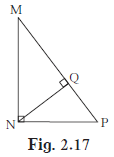In ∆MNP, ∠ MNP = 900,

By Pythagoras theorem,

MN2 + NP2 = MP2

⇒ MN2 + NP2 = (MQ + QP)2

⇒ MN2 + NP2 = (13)2

⇒ MN2 + NP2 = 169 … (1)

In ∆MQN, ∠MQN = 900,

QN2 + MQ2 = MN2

⇒ QN2 + 92 = MN2

⇒ QN2 + 81 = MN2 …(2)

In ∆PQN, ∠PQN = 900,

QN2 + PQ2 = PN2

⇒ QN2 + 42 = PN2

⇒ QN2 + 16 = PN2 … (3)

Now (2) + (3)

⇒ QN2 + 81 + QN2 + 16 = MN2 + PN2

⇒ 2QN2 + 97 = 169 [from(1)]

⇒ 2QN2 = 72

⇒ QN2 = 36

Thus NQ = 6.

Question 3.

In figure 2.18, ∠QPR = 90°, seg PM ⊥ seg QR and Q – M – R,PM = 10, QM = 8, find QR.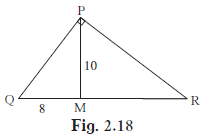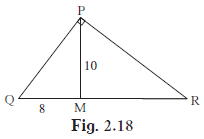In ∆PMQ, ∠PMQ = 900

So PM2 + QM2 = PQ2

⇒ 102 + 82 = PQ2

⇒ 100 + 64 =PQ2

PQ2 = 164 …(1)

In ∆PQR, ∠RPQ = 900

So PQ2 + PR2 = QR2

⇒ 164 + PR2 = QR2

⇒ PR2 = QR2 – 164 …(2)

In ∆PMR, ∠PMR = 900

So PM2 + MR2 = PR2

⇒ 102 + (QR – QM)2 = QR2 – 164

⇒ 100 + (QR – QM)2 = QR2 – 164

⇒ 100 + QR2 – 2.QR.QM + QM2 = QR2 – 164

⇒ 100 – 2.QR.8 + 64 = – 164

⇒ 16QR = 2×164

⇒ QR = 20.5

Thus QR = 20.5

Question 4.

See figure 2.19. Find RP and PSusing the information given in ΔPSR.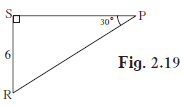Ans. RP = 12, PS = 6√3

In ∆PSR, ∠PSR = 900

So PS2 + SR2 = RP2

⇒ 62 + (RP cos(30°))2 = RP2

⇒ 62 + RP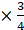= RP2

⇒ 62 =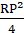⇒ RP2 = 4×36

Thus RP = 12.

PS = RP cos(300)

⇒ PS = 12×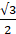PS = 6√3.

Question 5.

For finding AB and BC with the help of information given in figure 2.20, complete following activity.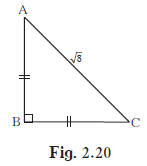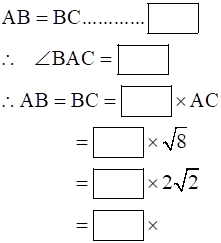In ∆ABC, ∠ABC = 900

So AB2 + BC2 = AC2

⇒ 2AB2 = 5

⇒ AB2 =⇒ AB = √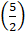= X(Say)

AB = BC = √∠BAC = 450 Since AB = BC

NOW √= X√5

X =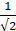Similarly, X2√2 = √X =Similarly, X√8 = √X =Question 6.

Find the side and perimeter of a square whose diagonal is 10 cm.

In a square of side say a cm, any diagonal divide the square into two right triangles of equal dimensions.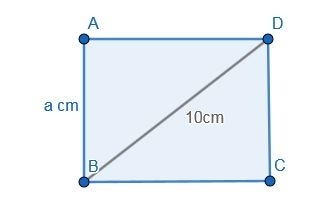Thus a2 + a2 = 102

⇒ 2a2 = 100

⇒ a2 = 50

a = 5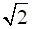cm

perimeter = 4a

= 4×5= 20Perimeter of square = 20cm

Question 7.

In figure 2.21, ∠DFE = 90°, FG ⊥ ED, If GD = 8, FG = 12,find (1) EG (2) FD and (3) EF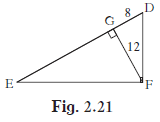In ∆DGF,DGF = 900

FD2 = DG2 + GF2

FD2 = 64 + 144

FD2 = 208

FD =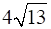In ∆DEF,DFE = 900

ED2 = DF2 + EF2

⇒ (EG + 8)2 = 208 + EF2 … (1)

In ∆EGF,FGE = 900

EF2 = EG2 + GF2

(EG + 8)2 – 208 = EG2 + 144

EG2 + 2.EG.8 + 64 – 208 = EG2 + 144 (As we know (a+b)2= a2+b2+2ab

EG = 18

From (1)

⇒ (EG + 8)2 = 208 + EF2

EF = 6√13.

Question 8.

Find the diagonal of a rectangle whose length is 35 cm and breadth is 12 cm.

The diagonal = √[length2 + breadth2]

= √(352 + 122)
= √(1225 + 144)

= √1369
= 37

Thus the diagonal is 37 cm.

Question 9.

In the figure 2.22, M is themidpoint of QR. ∠PRQ = 90°. Prove that, PQ2 = 4PM2 – 3PR2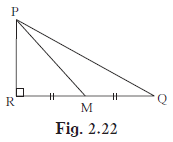In ∆PRQ, ∠PRQ = 900

PQ2 = PR2 + QR2 – – – 1

In ∆PRM, ∠PRM = 900

PM2 = PR2 + MR2

⇒ PM2 = PR2 +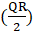2 [ M is midpoint]

⇒ 4(PM2 – PR2) = QR2 – – – 2

1 And 2 implies

PQ2 = PR2 + 4(PM2 – PR2)

⇒ PQ2 = 4PM2 – 3PR2

PROVED.

Question 10.

Walls of two buildings on either side of a street are parellel to each other. A ladder 5.8 m long is placed on the street such that its top just reaches the window of a building at the height of 4 m. On turning the ladder over to the other side of the street , its top touches the window of the other building at a height 4.2 m. Find the width of the street.

Let us consider a distance x m on the street from one building and a distance y m from the other one.

Now according to question,

In the 1st case,

5.82 = 42 + x2

⇒ x2 = 17.64

⇒ x = 4.2

Similarly for the second building,

5.82 = 4.22 + y2

⇒ y2 = 16

⇒ y = 4

Total width = x + y

= 4 + 4.2

= 8.2

Thus the total width is 8.2m.

## PDF FILE TO YOUR EMAIL IMMEDIATELY PURCHASE NOTES & PAPER SOLUTION. @ Rs. 50/- each (GST extra)

SUBJECTS

HINDI ENTIRE PAPER SOLUTION

MARATHI PAPER SOLUTION
SSC MATHS I PAPER SOLUTION
SSC MATHS II PAPER SOLUTION
SSC SCIENCE I PAPER SOLUTION
SSC SCIENCE II PAPER SOLUTION
SSC ENGLISH PAPER SOLUTION
SSC & HSC ENGLISH WRITING SKILL
HSC ACCOUNTS NOTES
HSC OCM NOTES
HSC ECONOMICS NOTES
HSC SECRETARIAL PRACTICE NOTES

2019 Board Paper Solution

HSC ENGLISH SET A 2019 21st February, 2019

HSC ENGLISH SET B 2019 21st February, 2019

HSC ENGLISH SET C 2019 21st February, 2019

HSC ENGLISH SET D 2019 21st February, 2019

SECRETARIAL PRACTICE (S.P) 2019 25th February, 2019

HSC XII PHYSICS 2019 25th February, 2019

CHEMISTRY XII HSC SOLUTION 27th, February, 2019

OCM PAPER SOLUTION 2019 27th, February, 2019

HSC MATHS PAPER SOLUTION COMMERCE, 2nd March, 2019

HSC MATHS PAPER SOLUTION SCIENCE 2nd, March, 2019

SSC ENGLISH STD 10 5TH MARCH, 2019.

HSC XII ACCOUNTS 2019 6th March, 2019

HSC XII BIOLOGY 2019 6TH March, 2019

HSC XII ECONOMICS 9Th March 2019

SSC Maths I March 2019 Solution 10th Standard11th, March, 2019

SSC MATHS II MARCH 2019 SOLUTION 10TH STD.13th March, 2019

SSC SCIENCE I MARCH 2019 SOLUTION 10TH STD. 15th March, 2019.

SSC SCIENCE II MARCH 2019 SOLUTION 10TH STD. 18th March, 2019.

SSC SOCIAL SCIENCE I MARCH 2019 SOLUTION20th March, 2019

SSC SOCIAL SCIENCE II MARCH 2019 SOLUTION, 22nd March, 2019

XII CBSE - BOARD - MARCH - 2019 ENGLISH - QP + SOLUTIONS, 2nd March, 2019

HSC Maharashtra Board Papers 2020

(Std 12th English Medium)

HSC ECONOMICS MARCH 2020

HSC OCM MARCH 2020

HSC ACCOUNTS MARCH 2020

HSC S.P. MARCH 2020

HSC ENGLISH MARCH 2020

HSC HINDI MARCH 2020

HSC MARATHI MARCH 2020

HSC MATHS MARCH 2020

SSC Maharashtra Board Papers 2020

(Std 10th English Medium)

English MARCH 2020

HindI MARCH 2020

Hindi (Composite) MARCH 2020

Marathi MARCH 2020

Mathematics (Paper 1) MARCH 2020

Mathematics (Paper 2) MARCH 2020

Sanskrit MARCH 2020

Important-formula

THANKS